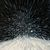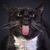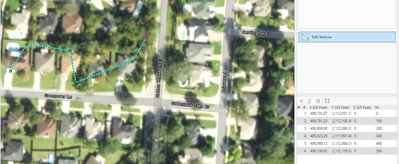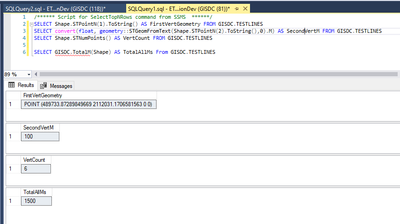# Get sum of M-values using SQL

360
4
04-08-2022 04:01 AM
Labels (1)by
Frequent Contributor

I have multipart polylines in an ST_GEOMETRY feature class (in an Oracle 18c EGDB).

Using SQL, I want to get the sum of the M-values of each polyline.

For example, for the following multipart line, the calculation would be: 11.18 + 33.54 + 43.54 = 88.26

(The M-sum is not to be confused with the length of the line.)How can I do that using SQL?

Tags (5)
4 Repliesby
Frequent Contributor

Reason: it’s a possible simplistic way to check M-values for issues:

Find polyline vertices where M-value is not cumulative length

The thought being: I could check the existing M-sum vs. the theoretical M-sum. If there is a difference, then that would tell me I have incorrect M-values.Occasional Contributor III

Not near a computer but I would think something like

Select sum(geometey.M) FROM FeatureTable GROUP BY PolylineID

But, like I said, haven't tested it and it in SQL Server speak 🙂by
Frequent Contributor

In SQL Server, does “geometey.M” generate individual rows for each vertex/M-value?

I’m not aware of a simple way to generate rows for each vertex…when it comes to SDE.ST_GEOMETRY in Oracle. We don’t have a GetVertices function, etc.Occasional Contributor III

Sorry.  You are correct.  That does not do what you want (should have tested it).

I had to write a customized function (I had to alter the original so ALTER rather than CREATE is shown):

``````ALTER FUNCTION [GISDC].[TotalM] (@geom geometry)
RETURNS FLOAT
WITH EXECUTE AS CALLER
AS
BEGIN
DECLARE @x integer;
DECLARE @y integer;
DECLARE @z float;
DECLARE @q float;
set @x = @geom.STNumPoints();
set @y = 1;
set @q = 0
WHILE @y <= @x
BEGIN
set @z = convert(float, geometry::STGeomFromText(@geom.STPointN(@y).ToString(),0).M);
set @q = @q + @z
set @y = @y + 1
END;
RETURN(@q);
END;
GO``````

Input test lineresult